GET THE APP

Empirical Performance Study of Alternative Option Pricing Models:# Journal of Stock & Forex TradingOpen Access

Research Article - (2013) Volume 2, Issue 2

# Empirical Performance Study of Alternative Option Pricing Models: An Application to the French Option Market

Sofiane Aboura*
Department of finance, University of Paris Dauphine, France
*Corresponding Author: Sofiane Aboura, Department of finance, University of Paris Dauphine, Place du Maréchal de Lattre de Tassigny, Paris Cedex 75775, France, Tel: 01 44 05 45 65 Email:

## Abstract

The mispricing of the deep-in-the money and deep-out-the-money generated by the Black and Scholes model is now well documented in the literature. In this paper, we discuss different option valuation models on the basis of empirical tests carry out on the French option market. We examine methods that account for non-normal skewness and kurtosis, relax the martingale restriction, mix two log-normal distributions, and allows either for jump diffusion process or for stochastic volatility. We find that the use of a jump diffusion and stochastic volatility model performs as well as the inclusion of non normal skewness and kurtosis in terms of precision in the option valuation.

<

Keywords: Implied Volatility, Stochastic Volatility Model, Jump Diffusion Model, Skewness, Kurtosis

#### Introduction

The failure of the Black and Scholes  model to provide correct valuation is attributable to the parsimonious assumptions used to derive the model. One of the strong assumptions of the model is that security prices follow a constant variance diffusion process with logreturns normally distributed whereas the constant variance assumption has always been rejected by many studies.

The ARCH models literature set up by Engle  and Bollerslev  has been devoted to the volatile behavior of stock return variances. This empirical literature has proved the stochastic nature of stock return variances and their correlation with security price levels shoing that returns are both skewed and leptokurtic. The systematic deviations from the observed prices correspond to a “gap” in the option valuation as noticed Black (1975) when stating that “one possible explanation for this pattern is that we have left something out of the formula”.

As Rubinstein  notice, the Black and Scholes  model tends to systematically misprice in-the-money and out-the-money options. Different studies lead to different conclusions knowing whether this model underprices deep-out-of-the-money and overprices deep-inof- the-money options or the contrary. Nevertheless, Rubinstein  concluded that the bias direction can change across different periods. The biases are not in the same direction for all markets and they are not constant over time. Observed moneyness biases constitute evidences against the hypothesis that asset returns are homoskedastic and normally distributed; this gave an impulse to the development of option pricing models for alternative processes.

The Constant Elasticity of Variance option pricing model of Cox and Ross  relax the constant volatility hypothesis and enabled the instantaneous conditional volatility of asset returns to depend deterministically upon the level of asset price. The implied binomial tree models of Dupire (1994), Derman and Kani (1994), and Rubinstein (1994) can be considered as flexible generalizations of the CEV model while the stochastic volatility option pricing models of Scott , Wiggins , Johnson and Shanno (1987) use numerical methods as the Monte Carlo simulations to price options when the variance varies.

Hull and White  solve explicitly for the options price by using Taylor expansion. But to produce analytical results they imposed the correlation between increments to be null. They solve the case for non zero correlation through Monte Carlo simulations. Hull and White  derive a model in series expansion form allowing for instantaneous correlation between stochastic volatility and the stock price.

Stein and Stein  derive a closed-form solution when volatility is driven by an Ornstein-Uhlenbeck (AR1) process but with zero correlation between both increments. Heston  proposes a closedform solution, but with arbitrary correlation between volatility and spot returns.

Jarrow and Rudd  approximate the log-normal probability distribution by an arbitrary distribution in terms of a series expansion. The idea is to derive an option pricing model expressed as the sum of the  formula plus adjustment terms permitting to capture the impact on the option price of the third and fourth moments of the underlying security stochastic process. In the same manner, Corrado and Su  derive and test empirically a European option pricing model that extends the  model to take into account for non-normal skewness and kurtosis in the distribution of stock returns.

The skewness and kurtosis coefficients are estimated simultaneously along with the implied standard deviation. They find that the adjustments for skewness and kurtosis are effective in removing systematic strike price biases from Black-Scholes  model for S&P500.

The recognition that asset returns are leptokurtic, especially for short periods, incited  to look for option pricing through jumpdiffusion processes. Ball and Torous [15,16] propose a simplified version of the jump-diffusion model known as the as the Bernoulli Jump process. Later on, Maltz  fitted this model to market option price data in order to estimate the ex-ante probability distribution of exchange rates. Bates  fit a jump diffusion option pricing model to option data extracting implicit volatility, skewness and kurtosis.

Duan  develops a GARCH option pricing model capable of reflecting the changes in the conditional volatility using numerical simulation. Ritchken and Trevor  developed also an option pricing model using a lattice algorithm to price both American and European options under discrete time GARCH processes. We recall that  showed that the GARCH model can be written as an approximation to certain diffusion equations which has been postulated in the option pricing literature. Duan, Gautier and Simonato  propose a series approximation to value American options for GARCH processes with one lag in the variance dynamics. Heston and Nandi  develop a closed-form option valuation formula for an asset whose variance follows a NGARCH process.

In this paper, we adopt Whaley’s  simultaneous equations procedure to estimate the implied parameters serving for the calculation of the option prices. This paper is organized as follows. Section 2 presents the models that are implemented. Section 3 gives details about sampling methodology. Section 4 displays the empirical results. Section 5 assesses the statistical performance of the models. Section 6 summarizes and concludes.

#### Option Pricing Models

We compare the out-of-sample performances of different option pricing models using the Black-Scholes  model as a benchmark.

The expansion methods are now largely used in quantitative finance. The idea is to start with an expansion formula for the risk neutral density considered as a general probability distribution. The first term of the expansion corresponds either to log-normal or normal distribution. The following terms can be therefore considered as successive corrections to the log-normal or normal approximations. The series is truncated at a finite order fixed empirically, which gives a parametric approximation of the risk neutral distribution. From this expression, we can derive an option pricing formula. The only drawback of this method is that when the infinite sum in the expansion represents a probability distribution, finite order approximations of it may become negative, leading to negative probabilities far enough in the tails, which generates severe mispricing for options that are far enough from the money.

We follow the approach of Corrado and Su  in the use of a semiparametric option pricing formula. They use the Gram-Charlier series expansion to model the distribution of stock log-prices. This method focuses on the skewness and kurtosis deviations from normality. Stuart and Ord (1987) discussed the distinction between Edgeworth expansion and Gram-Charlier expansion. To obtain an option pricing formula that correct the bias of the Black-Scholes  model, they add to this formula, adjustment terms accounting for non normal skewness and kurtosis. For a density function f(x), the Gram-Charlier series expansions are defined as: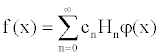φ(x) is a normal density function and H(x) are Hermite polynomials derived from successively higher derivatives of φ(x) while the coefficient Cn are determined by moments of the distribution function F(x). The Gram-Charlier series, which are infinite series, are here truncated to eliminate terms after the fourth moment. This turns out to be a good proxy for option pricing due to the consideration of non-normal skewness and kurtosis. A truncated series that take into account skewness and kurtosis gives the following density function with centered mean and reduced variance for the following density: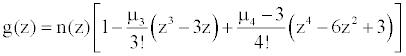with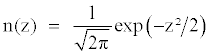Under risk-neutral measure, one can apply the density function g(z) to derive a formula for a European call as being the present value of an expected payoff at expiration. This call price is derived from the following expression: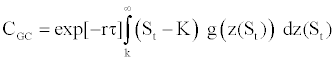where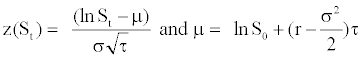We obtain an option pricing call formula based on the Gram- Charlier series as being: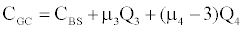where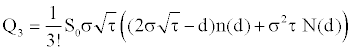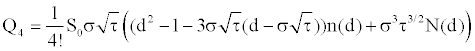with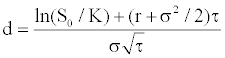The principle of the no-arbitrage approach is that the price of an option is obtained by taking the expectation of its discounting payoffs relative to a risk-neutral density. Arbitrage is not feasible in the condition that the risk-neutral density mean satisfy the martingale restriction. This restriction states that the price of the underlying asset implied by the option price must be the same that its observed market price, otherwise its should means the existence of arbitrage opportunities within a market with no frictions as shown by Harrisson and Kreps .

In the situation of frictions in the market, the option prices are determined by equilibrium and not by no-arbitrage reasoning, thus, the martingale restriction need not to be met. Among the frictions that can be encountered in the market, such as transaction costs or illiquidity, we can add information costs. The idea of estimating an implicit stock price is not recent. Manaster and Rendleman  is the first to invert  formula to estimate daily implied stock prices and daily implied volatility parameters. They concentrate their work around the idea of forecasting returns using the implied stock price.

They find that the implied stock price exceeds the observed price and affirmed that implied prices contain information regarding equilibrium stock prices that is not totally reflected in observed stock prices. One other reason to explain the difference between both stock prices was that transactions between both markets were not synchronous and thus, the difference represents more a recent rather than better information.

However, if they find that the second hypothesis explains a large part theses differences, “it is still possible that a significant fraction of implied prices represents more recent information than observed stock prices”. Later on, Longstaff  carries out a similar test on what he called “the martingale restriction”. He uses S&P100 index options data from 1988 to 1989, with American call options for which the American exercise effect should be alleviated. He focuses on a five minute window from 2:00 PM to 2:05 PM using index values during this window.

He find that the implied S&P100 index cost is superior to the actual index cost for 442 cases out of 444 daily estimates by an amount of 1.004. He explaines that since an option can be seen as a levered position in the underlying asset, which means that purchasing a stock through the option market costs more than on the spot market. We intuitively understand that accessing to a derivative market implies additive costs such as transaction or information costs.

Comparing the Black-Scholes  model with an equilibrium version of the model in which the martingale restriction is relaxed, he obtaines that, more than a half of the pricing errors occurring with the  model, is eliminated. We note that Patilea, Ravoteur and Renault (1995) propose an econometric approach based on the concept of implicit stock prices applied to the Hull and White  model and Renault  explains that introducing an implicit stock price may explain the skewness observed in the smile.

In this paper, we don’t test the martingale restriction following the very same methodology of Longstaff , we just want to check whether relaxing the martingale restriction, i.e, estimating an implicit stock price, will improve in our context the option pricing. We don’t limit ourselves to a small time period window since we don’t have enough observations per window.

Mixture of lognormal distributions

Melick and Thomas  estimate option pricing as a mixture of log-normal densities. They consider that the risk-neutral density can be adjusted correctly as a mixture of various log-normal densitites, representing different views of future reality. This method integers a number M of log-normal distributions but we limit our case to the simple case M=2. We suppose the risk-neutral density being equal to: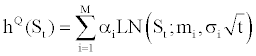with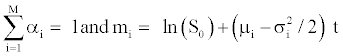and LN(St;mQ2t) is the log-normal density function under riskneutral measure. Within this configuration, the investors can imagine a situation for the future where, for instance, two configurations are expected, for which they affect two probabilities α1 and α2 and where the asset growth rate will be subject to two drift dynamics (μ1 and μ2) and two volatilities (σ1 and σ2). The call price of a mixture of log-normal densities can be written as: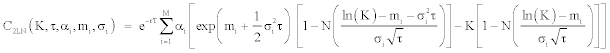Under the risk-neutral probability, we impose the following constraint: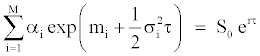The advantage of the procedure is that the option prices are given as an average of the Black-Scholes  prices for different volatilities weighted by the respective weights of each distribution in the mixture. By construction, this mixture of log-normals has thin tails unless one allows high values of variance. As recall Campa, Chang and Reider , probability in the tails declines monotonically and always decays quickly enough for preventing unrealistic kurtosis. The only drawback of this procedure is that it is not supported by any theoretical economic background, which could lead to a lack of economic meaning.

Stochastic volatility model

Hull and White  consider the following stochastic processes for the returns and for the volatility through the risk-neutral data generating process given by: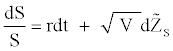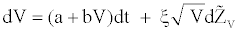where a, b, and ξ are constant and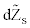and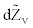are Wiener processes under risk-neutral probability and r is the instantaneous interest rate supposed constant. V is the asset’s instantaneous variance rate. In order to ensure that the drift rate of V will not be negative, it is required that a≥0. From the dynamic of the variance, we can obtain a constant drift with b=0, a constant proportional drift with a=0 or a mean reverting process with a>0 and b=0 from which V will tend to revert to a longrun level –a/b with a mean reversion rate –b. The time required for the expected deviation to be halved, the half-life is given by –ln2/b.

Hull and White  set up a power series expansion procedure based on the security price distribution conditional on the average value of the stochastic variance. This technique remains one of the most tractable one since if one compare it to the analytical approach proposed by Heston  based on Fourier inversion method. As in Corrado and Su , we set in the series expansion, the variance being equal to its long run reversion value since it is a credible hypothesis; however, running the model as it appears in the Hull and White  article is not more difficult, it simply requires an additional parameter to be estimated. Under this expansion, they showed how to correct the bias in the  model using a precise approximation from a second order Taylor series expansion:

Bias correction =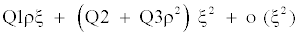The terms of the series expansions are defined as: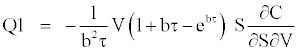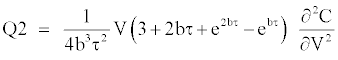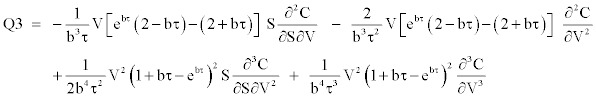We obtain a stochastic-adjusted call price when adding the bias correction to the Black and Scholes  call price, which yields: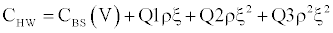If we assume a constant process variance, i.e, ξ = 0, we collapse in the Black and Scholes  formula since the pricing bias is null.

Jump diffusion model

The research of a distribution that fit the best the behavior of stock returns still continue to be a dominant issue in finance. After the introduction of arithmetic and geometric Brownian motions, much attention was devoted to Poisson distributions as a valid specification of stock returns. In addition to empirical evidence, these models succeeded in capturing the “abnormal” components of the total change in stock price as recalled Ball and Torous , while the “normal” component, considered as marginal changes, is captured by a standard log-normal diffusion process.

We know that large values of returns occur too often to be consistent with normality and also, positive and negative returns of a given size are not equally likely. We observe kurtosis if jumps in either direction are equally likely and we observe in addition skewness, if jumps in one direction are larger or more frequent. Both skewness and kurtosis are captured by the Poisson distribution as noticed Jorion . However, as recalled Renault , the expansion of these models are limited to the fact that there are few cases where closed-form solutions are given, specifically when there is a non zero probability of early exercise, or when the distribution of jumps is neither lognormal nor discrete. We follow Maltz  for pricing options with Jump Diffusion Model. In this section we assume that follows a log-normal jump diffusion, i.e., the addition of a geometric Brownian motion and a Poisson jump process. This price process under the risk-neutral probability can be shown to be: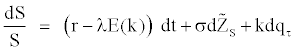with qτ a Poisson counter with average rate of jump occurrence λ (prob(dq = 1) = λdt) and k the jump size. [15-17] suppose as a simplification that during the life of the option there will occur at most one jump of constant size. If no events occur in the option life, the associated probability is (1–λτ) and will be λτ if one event occurs during this time interval. When such event occurs, there is an instantaneous jump in the stock price. This simplified version is called by Ball and Torous [15,16] as the Bernoulli distribution version of the jump-diffusion model: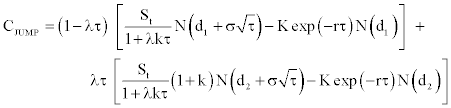where: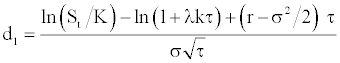and: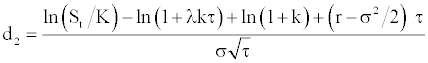This formula corresponds to the Black and Scholes  call option value weighted by the probability of a jump and by the probability of no jump with the stock price divided by the expected value of a jump (1–λkτ).

#### Data Description and Sampling Methodology

The description of the data

Option prices are extracted from SBF-BOURSE DE PARIS. The data set contains the PXL option quotations, the strike prices, the maturity and the CAC 40 quotations during October 1998. We use intra-day observations for all quotations. The PXL options are European style options that are written on the CAC 40 Index. Our study is based on call options. Their maturity lasts 6 month. The interest rate is taken from DATASTREAM. We chose the PIBOR 6 months as the risk-free interest rate. For the dividend yield, we use ex-post dividend rate downloaded from DATASTREAM.

The sampling methodology

We have in total 1402 intra-daily observations that represent option premium and all the features linked to these options, such as, the strike price, the underlying index price, the maturity, the minimum number of purchasable options, the risk-free interest rate. The maturity chosen is March 1999 and the minimum number of purchasable options is 50. The PIBOR interest rate is a daily rate.

To each option quote, correspond two intra-minutes CAC 40 quotes, since the index is quoted every 30 seconds, which means that there is a quotation for the first 30 seconds of a minute and another quotation for the last 30 seconds. We retain only the quotation pertaining to the first 30 seconds of the minute as underlying quote. We delete each quotation resembling exactly to the following.

There are 600 out-of-the-money calls and 28 in-the-money calls, i.e. 628 calls. There are 554 out-of-the-money puts and 220 in-themoney puts, i.e. 774 puts. For our study, we consider the 600 out-ofthe- money calls and the 554 out-of-the-money puts due to the fact they are more traded; they represent 1154 observations. We transform the out-of-the-money puts into theoretical observed in-the-money calls. Using the Black and Scholes  formula as a function, we extract the volatility by equating the function with the corresponding premium. Using this extracted volatility and the other corresponding parameters of the out-of-the-money puts, we re-compute through the Black and Scholes  formula for a call, a theoretical price of a supposed in-themoney call.

#### Empirical Tests

We don’t display the results showing the out-of-sample performance of the Black and Scholes  model as a benchmark. We first estimate daily implied standard deviation (ISD) of call options written on the CAC 40 index from intra-day data sample. We calculate a prior-day ISD and we use it as an input to compute the current-day option price. Theoretical Black and Scholes  prices based on an outof- sample ISD are then compared to their corresponding observed prices. The next models follow the same procedure.

First estimation procedure

We compute the Skewness and Kurtosis-adjusted Black and Scholes  option price model. We estimate the ISD, the option implicit skewness SK and the option implicit kurtosis KU, through the Simultaneous Equations Procedure, which gives us implied values of our parameters by minimizing the following sum of squares: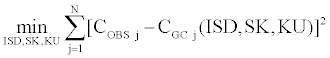CGC(ISD,SK,KU) is the call price of the proposed model computed with the ISD parameter and which includes the implied third and four moments SK, KU. This call price is calculated for any option in a given current day’s sample.

Column 7 gives the details about the results obtained by the comparison between the skewness and kurtosis-adjusted  model and the corresponding observed prices in the market. The average deviation spread in is computed as follows: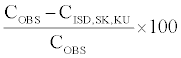Table 1 gives the results obtained from the first estimation procedure.

 Trading date Number of data ISD (%) Option Implied Skewness ISK Option Implied Kurtosis IKU Proportion of Theoretical Prices Different from the Observed Prices at ± 1 % Average Spread between Observed and Theoretical Price (%) Average Deviation of Theoretical Price from Observed Prices (FF) Average Call Price (FF) 02/10/98 153 49.416 -1.083 3.151 0.810 28.702 20.378 419.250 05/10/98 69 47.436 -1.062 2.831 0.696 1.909 17.839 528.873 06/10/98 53 45.694 -1.025 2.815 0.698 2.564 16.868 506.300 07/10/98 49 45.580 -1.195 3.712 0.673 19.961 20.444 406.673 08/10/98 56 46.754 -1.027 2.821 0.804 31.224 19.376 441.110 09/10/98 41 48.173 -1.447 4.203 0.659 -7.466 19.913 338.436 12/10/98 68 43.203 -1.196 2.883 0.985 -3.986 23.798 500.436 13/10/98 55 42.325 -1.253 2.894 0.400 1.082 18.696 626.313 14/10/98 86 42.859 -1.366 3.789 0.651 0.435 12.718 345.208 15/10/98 59 40.170 -1.195 2.907 0.864 11.065 16.228 378.210 16/10/98 60 39.765 -1.371 2.903 0.700 -5.325 14.172 385.422 19/10/98 32 39.941 -1.366 2.852 0.719 3.327 9.551 395.641 20/10/98 88 39.165 -1.289 2.916 0.580 7.274 11.190 526.652 21/10/98 57 40.007 -1.299 2.885 0.667 6.378 11.160 564.885 22/10/98 47 39.291 -1.330 2.893 0.532 -1.952 10.054 566.498 23/10/98 31 40.482 -1.269 2.827 0.903 24.462 15.179 391.574 26/10/98 25 38.303 -1.148 2.817 0.760 11.033 18.150 341.497 Average 64.111 42.857 -1.222 3.054 0.712 7.688 16.219 451.166

All the observed prices correspond to call options traded in October 1998 in the MONEP.

Table 1: Comparison of non normal skewness and kurtosis model prices and observed prices of PXL call options.

We notice that the average implied volatility is about 42.86% with a spike observed the second day reaching 49.41%. The average percentage obtained for the option implied skewness is -1.22. The average implied kurtosis is 3.05. We see in column six that in average, 71.2% of the theoretical prices are outside the ± 1% spread applied in the observed prices. Using the Black and Scholes  model, we obtain for the calls an average of 97.1%, which signifies a gain of about 26 %. The average deviation is around 16.22 FF (column 8) for an average call price of 451.16 FF (column 9). The average spread between the observed price and the corresponding theoretical price is about 7.68%, which indicates that the mispricing generally observed in the different models is significantly reduced with this model. Figure 1 shows the effects of allowing non-normal skewness and kurtosis in the theoretical ISD Black and Scholes  formula on October, 2nd 1998. We recall that positive moneyness signifies out-of-the-money options and negative moneyness implies in-the-money options. We see that this model reduces sharply the price deviation or bias effect observed in figure 1. This constitutes a clear improvement in terms of precision in the option valuation.

Second estimation procedure

We compute the implicit stock price in addition to the implied volatility from the Black and Scholes  model. We estimate the ISD, the option implied volatility and IS, the implicit underlying asset price, through the Simultaneous Equations Procedure, which gives us implied values of our parameters by minimizing the following sum of squares: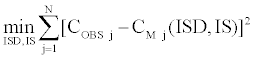CM(ISD,IS) is the call price of the second model computed with the ISD parameter and IS, the implied stock price. This call price is calculated for any option in a given current day’s sample (Table 2).

 Trading date Number of data ISD (%) Option Implied Stock Price IS Proportion of Theoretical Prices Different from the Observed Prices at ± 1 % Average Spread between Observed and Theoretical Price (%) Average Deviation of Theoretical Price from Observed Prices (FF) Average Call Price (FF) 02/10/98 153 38.158 3036.741 1.000 -60.031 76.532 419.250 05/10/98 69 37.506 3097.794 0.942 -19.283 45.895 528.873 06/10/98 53 37.433 3150.394 0.830 -16.927 50.194 506.300 07/10/98 49 34.885 3203.877 0.959 -44.953 33.987 406.673 08/10/98 56 37.989 3045.192 1.000 -68.087 79.396 441.110 09/10/98 41 30.986 3213.468 0.976 -11.808 54.859 338.436 12/10/98 68 32.368 3316.699 1.000 14.847 72.750 500.436 13/10/98 55 33.166 3339.479 0.836 -1.775 39.135 626.313 14/10/98 86 28.806 3422.876 0.919 -8.973 32.703 345.208 15/10/98 59 30.550 3457.335 0.847 -6.200 33.062 378.210 16/10/98 60 29.257 3496.891 0.900 -2.293 24.723 385.422 19/10/98 32 29.516 3492.022 0.938 -9.126 22.979 395.641 20/10/98 88 32.620 3477.614 0.898 -3.893 32.953 526.652 21/10/98 57 32.520 3492.155 0.947 -12.402 31.770 564.885 22/10/98 47 33.129 3503.112 0.915 -8.276 36.129 566.498 23/10/98 31 28.902 3500.633 0.968 -49.638 36.298 391.574 26/10/98 25 30.023 3529.909 0.960 -6.669 29.141 341.497 Average 64.111 33.035 3339.776 0.931 -18.558 43.089 451.166

All the observed prices correspond to call options traded in October 1998 in the MONEP.

Table 2: Comparison of implicit stock price model and observed values of PXL call options.

We notice that the average implied volatility is about 33.03% with a spike observed the second day reaching 38.16%. The average percentage obtained for the option implied stock price is 3339.77. We see in column five that in average, 93.1% of the theoretical prices are outside the ± 1% spread applied in the observed prices. Three of them are completely outside, which indicates a very slight improvement, but that is not significant relative to our benchmark, i.e., the Black and Scholes  model. The average deviation is around 43.09 FF in column 7 for a average call price of 451.16 FF. The average spread between the observed price and the corresponding theoretical price is about -18.56%, which indicates that the mispricing generally observed in the benchmark is reduced by two. Figure 2 shows the effects of estimating implicitly the stock price in the theoretical Black and Scholes  formula on October, 2nd 1998. We globally see that options around the money are generally all over-estimated. The estimation failed to provide an improvement in terms of price deviation. 16 out of 18 daily implicit stock prices are superior to the observed stock prices and the average difference between them is about 2.27% with a median value at 2.54% and about 2.50% when compared to the average of observed values in the day.

Third estimation procedure

In this procedure, we compute an option price as a mixture of two log-normal distributions. We estimate two implied volatilities and m1, m2, α1, α2 through the Simultaneous Equations Procedure, which gives us implied values of the parameters. The Simultaneous Equations Procedure minimizes the following sum of squares: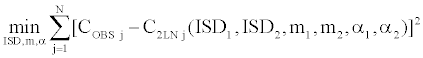C2LN(ISD1, ISD2, m1, m2, α1, α2) is the call price of the proposed model computed for any option in a given current day’s sample (Table 3).

 Trading date ISD1 (%) ISD2 (%) m1 m2 α1 Proportion of Theoretical Prices Different from the Observed Prices at ± 1 % Average Spread between Observed and Theoretical Price (%) Average Deviation of Theoretical Price from Observed Prices (FF) 02/10/98 36.503 39.046 8.047 8.042 0.848 1.000 -60.557 76.632 05/10/98 46.494 10.486 7.841 8.236 0.685 0.986 5.865 39.746 06/10/98 26.979 41.575 8.211 7.854 0.404 1.000 -18.158 41.204 07/10/98 19.579 68.446 8.187 7.595 0.606 0.918 -18.724 27.639 08/10/98 34.642 33.996 8.070 8.013 0.834 1.000 -66.727 79.382 09/10/98 41.005 11.800 7.889 8.250 0.725 0.976 10.982 40.351 12/10/98 30.449 32.399 8.079 8.024 0.827 0.971 15.293 75.205 13/10/98 23.065 45.590 8.196 7.776 0.685 0.945 3.711 33.933 14/10/98 31.404 43.655 8.113 8.045 0.862 0.930 -8.326 31.660 15/10/98 26.341 45.359 8.157 7.968 0.837 0.864 -6.018 32.795 16/10/98 17.729 55.596 8.257 7.736 0.652 0.817 5.180 15.740 19/10/98 44.034 18.006 7.947 8.260 0.441 0.875 -4.066 14.355 20/10/98 45.498 16.593 7.898 8.271 0.442 0.955 7.003 30.265 21/10/98 51.874 16.550 7.890 8.269 0.419 0.526 -1.264 15.680 22/10/98 45.592 17.366 7.916 8.275 0.424 0.872 4.011 22.267 23/10/98 47.876 19.694 7.909 8.266 0.390 1.000 -26.642 42.918 26/10/98 19.857 17.260 7.950 8.286 0.411 0.960 11.267 23.085 Average 50.495 15.995 7.961 8.256 0.425 0.917 -8.657 37.815

All the observed prices correspond to call options traded in October 1998 in the MONEP.

Table 3: Comparison of mixture of lognormal distribution model and observed values of PXL call options.

We notice that the average implied volatility is about 50.50% for ISD1 and three times less for ISD2 with 16%. We see in column seven that in average, 91.7% of the theoretical prices are outside the ±1% spread applied to the observed prices. Four of them are completely outside, which indicates a slight improvement, but that is not significant relative to our benchmark.

The average deviation is around 37.81 FF in column 9 for an average call price of 451.16 FF. The average spread between the observed prices and the corresponding theoretical prices is about -8.66%, this is an improvement in pricing compared to the benchmark (Figure 3) shows the effects of considering a mixture of two log-normal distributions on October, 2nd 1998.

This form of estimation failed to provide an improvement in terms of price deviation and we observe that the prices generated by this mixture are globally the same than those generated by the implicit stock price model.

Fourth estimation procedure

We estimate implicitly the parameters of the Hull and White  model. We estimate the correlation parameter ρ, the volatility of volatility parameter ξ and the coefficient of mean reversion b and the ISD through the Simultaneous Equations Procedure, which gives us implied values of our parameters by minimizing the following sum of squares: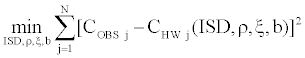CHW(ρ, ξ, b) is the call price of the Hull and White  model. This call price is calculated for any option in a given current day’s sample (Table 4).

 Trading date Number of Data ISD (%) Implied Volatility of Volaility Implied Correlation Half-Life Of Volatility (Days) Proportion of Theoretical Prices Different from the Observed Prices at  ± 1 % Average Spread between Observed and Theoretical Price (%) Average Deviation of Theoretical Price from Observed Prices (FF) 02/10/98 153 44.397 2.655 -0.560 21.357 0.974 35.785 30.525 05/10/98 69 43.575 2.101 -0.696 21.229 0.913 16.722 25.472 06/10/98 53 41.858 2.994 -0.520 21.443 0.943 13.139 19.630 07/10/98 49 41.391 2.241 -0.624 21.253 0.898 33.809 21.958 08/10/98 56 42.251 3.156 -0.478 21.456 1.000 52.034 28.610 09/10/98 41 41.497 1.856 -0.717 21.165 1.000 0.751 20.252 12/10/98 68 40.347 2.531 -0.629 21.333 0.897 3.734 25.566 13/10/98 55 40.843 3.549 -0.460 21.563 0.818 5.202 22.714 14/10/98 86 37.122 4.496 -0.421 21.795 0.860 6.620 15.132 15/10/98 59 35.363 2.082 -0.715 21.224 0.966 44.622 25.641 16/10/98 60 35.043 1.699 -0.655 21.127 0.983 0.208 21.515 19/10/98 32 36.232 2.337 -0.652 21.278 0.938 10.951 23.923 20/10/98 88 35.031 2.936 -0.526 21.415 0.932 12.970 22.693 21/10/98 57 36.609 2.577 -0.516 21.316 0.947 12.752 27.162 22/10/98 47 35.676 3.063 -0.495 21.437 0.872 2.533 18.458 23/10/98 31 37.675 2.873 -0.512 21.392 0.968 34.398 26.174 26/10/98 25 34.912 3.101 -0.486 21.445 0.920 17.998 20.984 Average 64.111 39.038 1.858 -0.606 21.157 0.931 17.896 23.318

All the observed prices correspond to call options traded in October 1998 in the MONEP

Table 4: Comparison of implicit Hull and White (1988) model and observed values of PXL call options.

The average percentage obtained for the implied correlation parameter is –0.60. We see in column five that in average, 93.1% of the theoretical prices are outside the ± 1% spread applied to the observed prices. Two of them are completely outside, which indicates a very slight improvement, but that is not significant relative to our benchmark. The average deviation is around 23.32 FF in column 9 for a average call price of 451.16 FF. The average spread between the observed price and the corresponding theoretical price is about 17.89%, which shows that the mispricing generally observed in the benchmark is reduced two times. (Figure 4) shows the effects of estimating option prices with a stochastic volatility model on October, 2nd 1998.

The Hull and White  model provides significant improvements in terms of price deviations for far from the money call options.

Fifth estimation procedure

We estimate implicitly the parameters of the jump diffusion model. We estimate the jump occurrence parameter λ, the jump size parameter k and the implied volatility through the Simultaneous Equations Procedure, which gives us implied values of three parameters by minimizing the following sum of squares: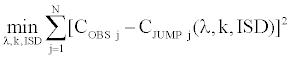CJUMP(λ, k, ISD) is the call price given by the jump diffusion model. This call price is calculated for any option in a given current day’s sample (Table 5).

 Trading date Number of data ISD Option Implied Jump Occurrence Option Implied Jump Size Proportion of Theoretical Prices Different from the Observed Prices at ± 1 % Average Spread between Observed and Theoretical Price (%) Average Deviation of Theoretical Price from Observed Prices (FF) Average Call Price (FF) 02/10/98 153 0.273 0.377 -0.537 0.778 4.490 17.148 419.250 05/10/98 69 0.279 0.421 -0.537 0.768 -13.107 16.414 528.873 06/10/98 53 0.269 0.387 -0.544 0.642 -6.343 14.829 506.300 07/10/98 49 0.272 0.359 -0.542 0.857 -17.044 16.482 406.673 08/10/98 56 0.259 0.389 -0.509 0.875 6.844 17.806 441.110 09/10/98 41 0.265 0.397 -0.538 0.805 -9.838 15.616 338.436 12/10/98 68 0.237 0.517 -0.473 0.926 -6.087 19.867 500.436 13/10/98 55 0.254 0.382 -0.498 0.582 -3.704 17.429 626.313 14/10/98 86 0.254 0.341 -0.510 0.709 -5.438 10.354 345.208 15/10/98 59 0.233 0.421 -0.471 0.763 -12.297 11.351 378.210 16/10/98 60 0.225 0.415 -0.467 0.767 -3.076 10.705 385.422 19/10/98 32 0.227 0.397 -0.463 0.563 -1.906 7.855 395.641 20/10/98 88 0.211 0.477 -0.440 0.693 -1.053 19.192 526.652 21/10/98 57 0.237 0.335 -0.492 0.596 -1.349 17.138 564.885 22/10/98 47 0.254 0.270 -0.536 0.574 -5.698 15.451 566.498 23/10/98 31 0.249 0.268 -0.533 0.871 -5.348 14.571 391.574 26/10/98 25 0.214 0.516 -0.427 0.920 -6.417 15.680 341.497 Average 64.111 0.239 0.297 -0.529 0.746 -5.139 15.170 451.166

All the observed prices correspond to call options traded in October 1998 in the MONEP.

Table 5: Comparison of jump diffusion model prices and observed prices of PXL call options.

We notice that the average implied volatility is about 24 %. The average percentage obtained for the implied average rate of jump occurrence is 0.297 and the implied average jump size is about –0.53. About 74.62% of the theoretical prices are lying outside the ±1% spread applied to the observed prices. No one is totally outside, showing that the improvements that brings this jump diffusion model.

The total gain relative to the benchmark is about 23 %. The average deviation is around 15.17 FF for an average call price of 451.16 FF. The average spread between the observed prices and the corresponding theoretical prices is about –5.14 %, which indicates a considerable decrease in the mispricing. Figure 2 shows the effects of allowing one jump of constant size in the theoretical  framework on October, 2nd 1998 (Figure 5).

The reduction in the price deviation is clear comparing to the benchmark and is very comparable to model 2 and model 5.

#### The Statistical Performance of the Models

We want to evaluate the statistical significance of the improvement in terms of performance from out-of-sample adjustments brought by each model and for that, we use the following Z-statistic that represents the difference between the two proportions, P1 and P2 Hoel (1984):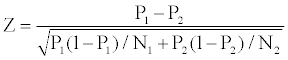P1 and P2 are sample proportions, while N1 and N2 are corresponding sample sizes.

We obtain after computation:

For the first model: Z=18.218 - P1=0.971 and P2=0.712; N1=N2= 1154

For the second and fourth model: Z=4.470 - P1=0.971 and P2=0.93; N1=N2=1154

For the third model: Z=5.680 - P1=0.971 and P2=0.917; N1=N2=1154

For the fifth model: Z=16.383 - P1=0.971 and P2=0.746; N1=N2=1154

The Z-statistics of 4.470, 5.680 and 18.218 are statistically significant at more than 99.99% confident level, which corresponds to a fractile of 2.32.

We want to go further into the analysis of the out-of-sample performance of our various models. We denote C, the observed price of the option and C , the theoretical price of the same option. We use the Mean of Absolute forecast Error (MAE) and the Mean Absolute Percentage forecast Error (MAPE) (Lauterbach and Schultz (1990)). For n being the number of options, the value of the MAE is equal to the mean of the difference in absolute value between the observed prices and the theoretical prices: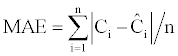For each option the valuation error percentage is given by: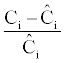The MAPE value is: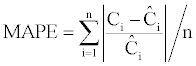The value of MAE and MAPE are actually complementary because if the first one gives a classical estimation of the difference, the second one gives the difference relative to the theoretical price. Tables 6 and 7 give the results obtained using the PE, MAE and MAPE measures (Table 6).

 Models MAE MAPE PE Benchmark 38.827 0.209 1.206 I - Series expansions 11.248 0.413 -1.668 II - Martingale restriction 40.760 0.171 -1.312 III - Mixture of log-normals 36.818 0.146 0.030 IV - Stochastic volatility 18.589 0.628 10.497 V - Jump diffusion 10.007 0.0632 -0.899

The pricing errors are computed as the difference between the observed call price and the theoretical price.

Table 6: Comparison between alternative models.

 Models MAE MAPE PE ITM OTM ITM OTM ITM OTM Benchmark 41.673 36.271 0.061 0.342 42.806 -36.349 I - Series expansions 9.785 12.563 0.014 0.772 -0.514 -4.783 II – Martingale restriction 60.235 23.262 0.0869 0.247 28.734 -12.489 III – Mixture of log-normals 55.285 20.225 0.074 0.211 20.146 -1.045 IV – Stochastic volatility 22.418 15.149 0.03 1.166 21.717 0.336 V - Jump diffusion 12.24 8 0.0165 0.105 -0.907 -4.389

Table 7: Comparison between alternative models for ITM and OTM call options.

The jump diffusion model holds the best MAPE and MAE and the second best pricing errors while the stochastic volatility model has surprinsingly the worst MAPE and pricing errors. (Table 7) presents the forecast errors distinguishing in-the-money options and out-themoney options.

We observe that the series in expansion model produces the best forecasts concerning ITM options for PE, MAE and MAPE measures. However, the best MAE and MAPE measures concerning OTM options, are obtained by the jump diffusion model, which has also the second best pricing error measure. The martingale restriction model has the worst performance among the five other models. The two models (1 and 5) that overestimate the ITM options have the best PE; the only model (4) that underestimates the OTM options has also the best PE. We globally note that the stochastic volatility model and the series in expansions model suffer form severe mispricing of OTM options.

#### Conclusion

In this paper, we carry out empirical tests of different models likely to correct the pricing bias that occurred in the classical Black and Scholes  framework and we also test the statistical performance of the given models. We observed that the Black and Scholes  model undervalues out-the-money calls and overvalues in-the-money calls. The jump diffusion model performs better than the other four models and overall has the best results concerning the out-the-money call options while the series in expansion model holds the best forecasts concerning the in-the-money call options. The stochastic volatility model arrives in third position with no outstanding performances. As for the mixing two log-normal distibutions and the implicit stock price model, they didn’t offer the expected precision. In further research, we will extend this work to propose an option valuation model that incorporates both transaction costs and information costs in order to correct the bias due to the frictions in the market.

#### References

1. Black F, Scholes M (1973) The Pricing of Options and Corporate Liabilities. J Polit Econ 81: 637-654.
2. Engle RF(1982) Autoregressive Conditional Heteroskedasticity with Estimates of the Variance of U.K Inflation. Econometrica 50: 987-1008.
3. Bollerslev T (1986) Generalized Autoregressive Conditional Heteroskedasticity. J Econometrics 31: 307-327.
4. Rubinstein M (1985) Nonparametric Tests of Alternative Option Pricing Models using all Reported Traded and Quotes on the 30 Most Active CBOE Option Classes from August 23, 1976 through August 31, 1978. J Financ 40: 455-480.
5. Cox JC, Ross SA (1976) The Valuation of Options for Alternative Stochastic Processes. J Financ Econ 3: 145-66.
6. Scott LO (1987) Option Pricing when Variance Changes Randomly: Theory Estimation and an Application. J Financ Quant Anal 22: 419-438.
7. Wiggins JB (1987) Option Values under Stochastic Volatility: Theory and Empirical Estimates. J Financ Econ 19: 351-372.
8. Hull J, White A (1987) The Pricing of Options on Assets with Stochastic Volatility. J Financ 42: 281-300.
9. Hull J, White A (1988) An Analysis of the Bias in Option Pricing Caused by Stochastic Volatility. Advances in Futures and Options Research, JAI Press.
10. Stein EM, Stein JC (1991) Stock Price Distribution with Stochastic Volatility: An Analytical Approach. Rev Financ Stud 4: 727-752.
11. Heston S (1993) A Closed Form Solution for Options with Stochastic Volatility with Applications to Bond and Currency Options. Rev Financ Stud 6: 327-344.
12. Jarrow R, Rudd A (1982) Approximate Option Valuation for Arbitrary Stochastic Process. J Financ Econ 10: 347-369.
13. Corrado CJ, Su T (1996) Skewness and Kurtosis in S&P 500 Index Returns Implied by Option Prices. J Financ Res 2: 175-192.
14. Merton RC (1976) Option Pricing When Underlying Stock Returns are Discontinuous. J Financ Econ 3: 125-144.
15. Ball CA, Torous WN (1983) A Simplified Jump Process for Common Stock Returns. J Financ Quant Anal 18: 53-65.
16. Ball CA, Torous WN (1985) On Jumps in Common Stock Prices and their Impact on Call Option Pricing. J Financ 40: 155-173.
17. Maltz AM (1996) Using Option Prices to Estimate Realignment Probabilities in the European Monetary System : The Case of Sterling–Mark. J Int Money Financ 15: 717-745.
18. Bates DS (1996) Jumps and Stochastic Volatility: Exchange Rate Processes Implicit in Deutsche Mark Options. Rev Financ Stud 9: 69-107.
19. Ritchken P, Trevor R (1999) Pricing Options under Generalized GARCH and Stochastic Volatility Processes. J Financ 1: 377-400.
20. Nelson DB (1990) ARCH Models as Diffusion Approximations. J Econometrics 45: 7-38.
21. Duan JC, Gautier G, Simonato J (1999) An Analytical Approximation for the GARCH Option Pricing Model. Journal of Computational Finance 2: 75-116.
22. Heston S, Nandi S (2000) A Closed-Form GARCH Option Valuation Model. Rev Financ Stud 13: 585-625.
23. Whaley RE (19820 Valuation of American Call Options on Dividend Paying Stocks. J Financ Econ 10: 29-58.
24. Harrisson JM, Kreps DM (1979) Martingale and Multiperiod Securities Markets. J Econ Theory 20: 381-408.
25. Manaster S, Rendleman R (1982) Option Prices as Predictors of Equilibrium Stock Prices. J Financ 37: 1043-1057.
26. Lonstaff AF (1995) Option Pricing and Martingale Restriction. Rev Financ Stud 8: 1091-1124.
27. Renault E (1998) Alternatives to the Black-Scholes Option Pricing Model and When to Use Them. Working Paper.
28. Melick A, Thomas CP (1997) Recovering an Asset’s Implied PDF from Options Prices: An Application to Crude Oil during the Gulf Crisis. J Financ Quant Anal 32: 91-116.
29. Campa JM, Chang PHK, Reider RL (1998) Implied Exchange Rate Distributions Evidence from OTC Option Markets. J Int Money Financ 17: 117-160.
30. Jorion P (19880 On Jump Processes in the Foreign Exchange and Stock Markets. Rev Financ Stud 1: 427-445.
Citation: Aboura S (2013) Empirical Performance Study of Alternative Option Pricing Models: An Application to the French Option Market. J Stock Forex Trad 2:108.

Copyright: © 2013 Aboura S. This is an open-access article distributed under the terms of the Creative Commons Attribution License, which permits unrestricted use, distribution, and reproduction in any medium, provided the original author and source are credited.
Top